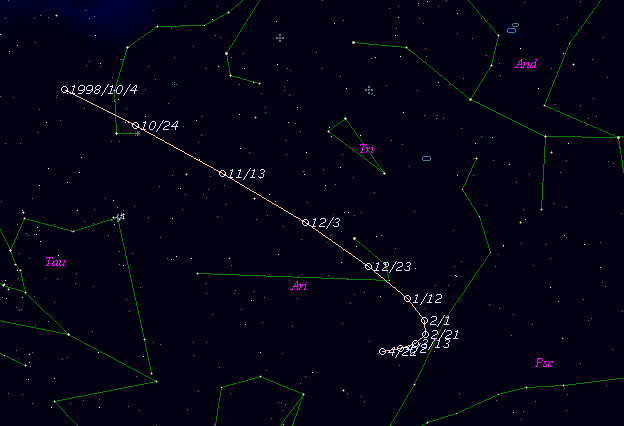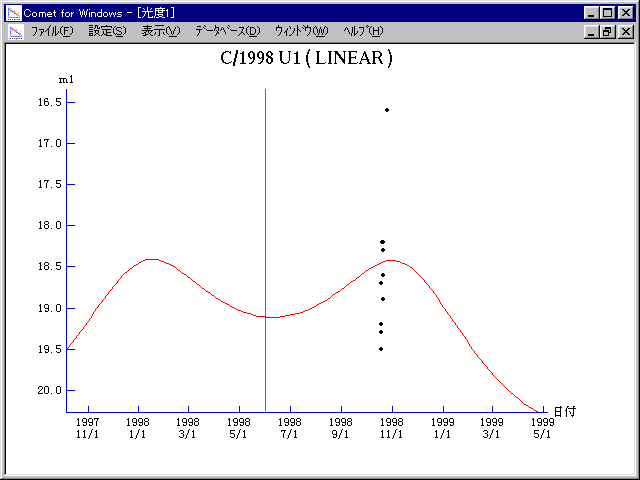# \$B%j%K%"WB@1(B

C/1998 U1 ( LINEAR )###\$B%W%m%U%#!<%k(B

 \$BH/8+F|(B 1998\$BG/(B10\$B7n(B18\$BF|(B \$BH/8+8wEY(B 19.2\$BEy(B \$BH/8+ Lincoln Laboratory Near-Earth Asteroid Research project

###\$B50F;MWAG(B

```   The following improved orbital elements by Kenji Muraoka, are
from 113 observations 1998 Oct. 18 to 1999 Jan. 13, perturbations
by 9 Planets, Moon and 5 minor planets were taken into account.
The mean residual is +/- 0.76 arc seconds.

Epoch  =  1998 Apr. 17.0  TT       JDT = 2450920.5
T  =  1998 May   2.41876       +/- 0.05503 (m.e.) TT
Peri. =  124.62945                +/- 0.01046
Node  =  210.45528                +/- 0.01228   (2000.0)
Incl. =  156.42896                +/- 0.00310
q  =    3.9752022              +/- 0.0002207 AU
e  =    1.0005227              +/- 0.0004884
1/a  =   -0.0001315              +/- 0.0001229 1/AU
```

###\$B@1?^(B###\$B8wEYJQ2=(B

```        m1 = 9.5 + 5 log\$B&\$(B + 10.0 log r
```##### \$B50F;MWAG\$OB<2,7r<#;a\$N7W;;\$K\$h\$k\$b\$N\$G\$9!#(B \$B@1?^\$O(B StellaNavigator Ver.2.0 for Windows (\$B%"%9%H%m%"!<%D(B \$BJTCx(B / \$B%"%9%-!<=PHG6I4)(B) \$B\$G:n@.\$7\$?\$b\$N\$G\$9!#(B \$B8wEY%0%i%U\$O(BComet for Windows\$B\$G:n@.\$7\$?\$b\$N\$G\$9!#(B